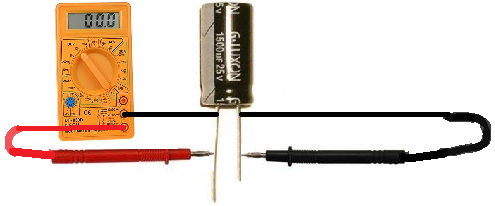﻿ How to Measure Capacitance

# How To Measure CapacitanceIn this article, we will go over one main way to measure the capacitance of a capacitor or any device, which is by using a capacitance meter. This may be a standalone capacitance meter or part of a multimeter.

Measure Capacitance with a Capacitance Meter of a Multimeter

The best and easiest way to measure capacitance is through a multimeter which contains a capacitance meter.

Simply switch the meter to the capacitance meter and take the probes and measure across the leads of the capacitor.This will enable you to read the capacitance.

Related Resources